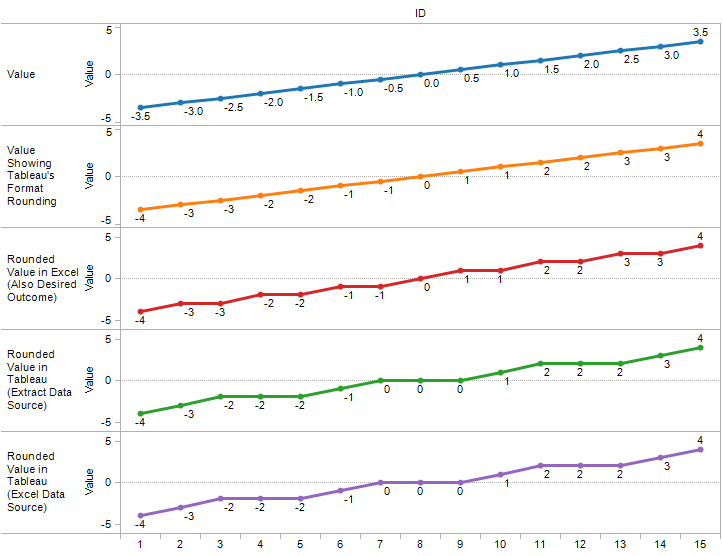# Unexpected Results: Rounding

When using Tableau with different data sources, it becomes obvious fairly quickly that there are differences in what functions are available in one data source vs. another. For example, MEDIAN() and COUNTD() are functions not available in MS Excel, Access, or text data sources, but are available in Tableau Data Extracts and many others. This post goes into a case where the same function is available, but is returning different results than we might expect depending on context, and introduces a workaround.

The function is ROUND(),  and the rounding using Tableau’s built in number formatting. The number rounding we get taught as kids (in the USA at least) is known as “round half away from zero,” that is, we round to the next bigger absolute number, so 1.5 gets rounded to 2 and -1.5 gets rounded to -2. This is what Microsoft Excel uses, as we can see here: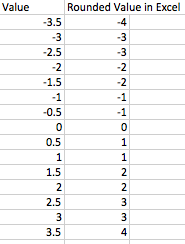## Number Formatting Works Differently Depending on View

In Tableau, if we set the number format by right-clicking a numeric Measure or Dimension and choose Field Properties->Number Format…, or right-clicking on a pill in the view to choose Format->[field name]->Header or Pane Tab->Numbers, we’ll also see round half away from zero: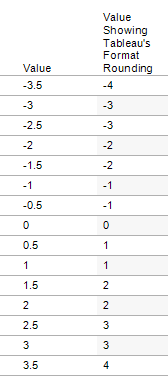However, that format rounding is only for display in text tables and mark labels. Here’s a line graph demonstrating the difference.

• The Value line uses the original data on both the line and the mark labels
• The Value Showing Tableau’s Format Rounding uses the original data for the line, but uses the formatted (rounded) number for the Mark Label and the two are different. Note that this happens for other Mark Types as well.
• The Rounded Value in Excel line shows the desired outcome, where both the line and the mark label show the same rounding.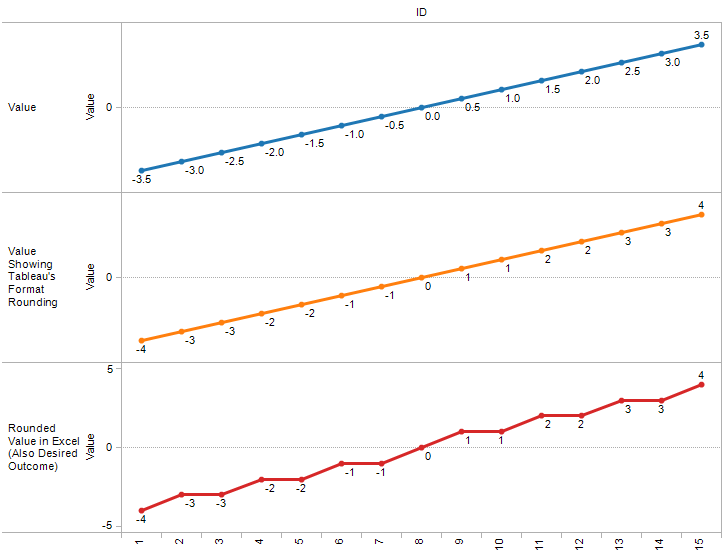## ROUND() Works Differently from Number Formatting

To generate a rounded number in Tableau, we turn to the ROUND() function. Here’s a line graph using the ROUND() function on the same data as above, adding lines for the results of ROUND() from an Excel file and a Tableau Data Extract. The formula for each is ROUND([Value],0). Compare the last three lines, and see some differences:For the last two lines, there are three marks with values of -2, 0, and 2, instead of two, one, and two marks as in the Rounded Value in Excel line. We can also see this in a text table: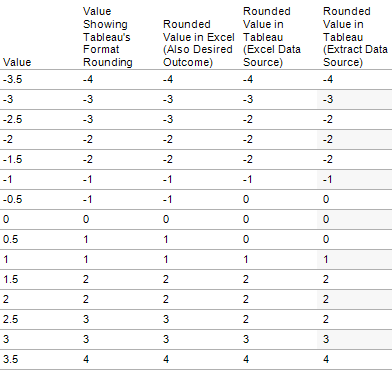What’s happening here is that Tableau is using bankers’ rounding, also known as “round half to even.” A number like -0.5 is rounded to the next nearest even number, so it goes to 0, while 1.5 goes to 2. The reason why Tableau does this is that bankers’ rounding is generally more accurate than the round half away from zero rule. Note that the Tableau documentation on the ROUND() function (as of 10/14/12) does not describe this, and instead just says “…rounded to the nearest integer.”:

### ROUND(number, [decimals])

Rounds numbers to a specified number of digits. The decimals argument specifies how many decimal points of precision to include in the final result, and it is not required. If the decimals variable is omitted, number is rounded to the nearest integer. For example, ROUND(7.3) = 7 ROUND(-6.9) = -7 ROUND(123.47,1) = 123.5 ROUND(Sales) rounds every Sales value to an integer.

Note that some databases such as MS SQL Server, allow specification of a negative length, where -1 rounds number to 10’s, -2 rounds to 100’s, and so on. This is not true of all databases to which you can connect. For example, it is not true of Excel or Access.

In my case, I needed to round half away from zero in order to match results coming from a legacy system. So, what can we do to get a round half away from zero number? While interacting with Tableau tech support on this issue, they provided me with a formula, that with a slight revision works perfectly:

`ROUND(IF [Value] > 0 THEN [Value]+0.000001 ELSE [Value]-0.000001 END,0)`

What the formula does is make sure that each value is made just a little bit bigger for positive .5, or just a little bit smaller for -.5, so the rounding is to the nearest. If you are dealing with large numbers of decimal places, add a few 0’s to the number to make sure that the bankers’ rounding is not triggered.

Here’s a line graph showing the formula providing the same results for both Excel and Tableau Data Extract data sources: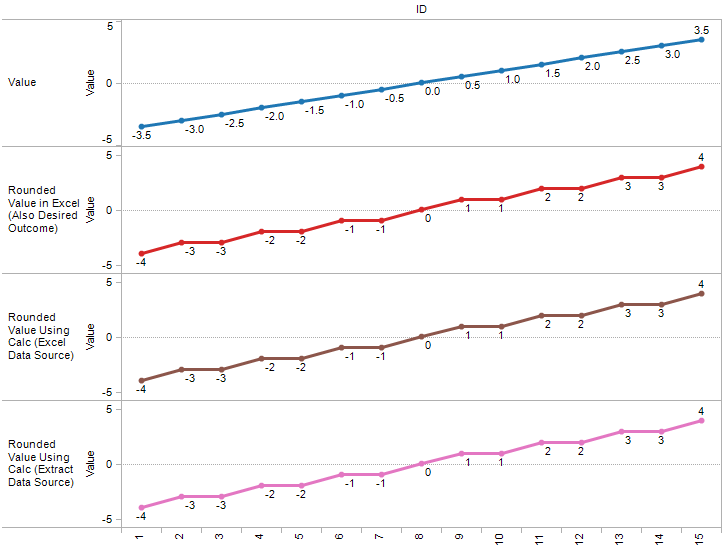Thanks to Tableau tech support for their help on this, I’ve also asked them to add clarification to the Tableau documentation.

## 2 thoughts on “Unexpected Results: Rounding”

1.Shawn Wallwork

Jonathan, nicely written. Very informative, I’d never even heard of “Banker’s Rounding”. Thx

–Shawn